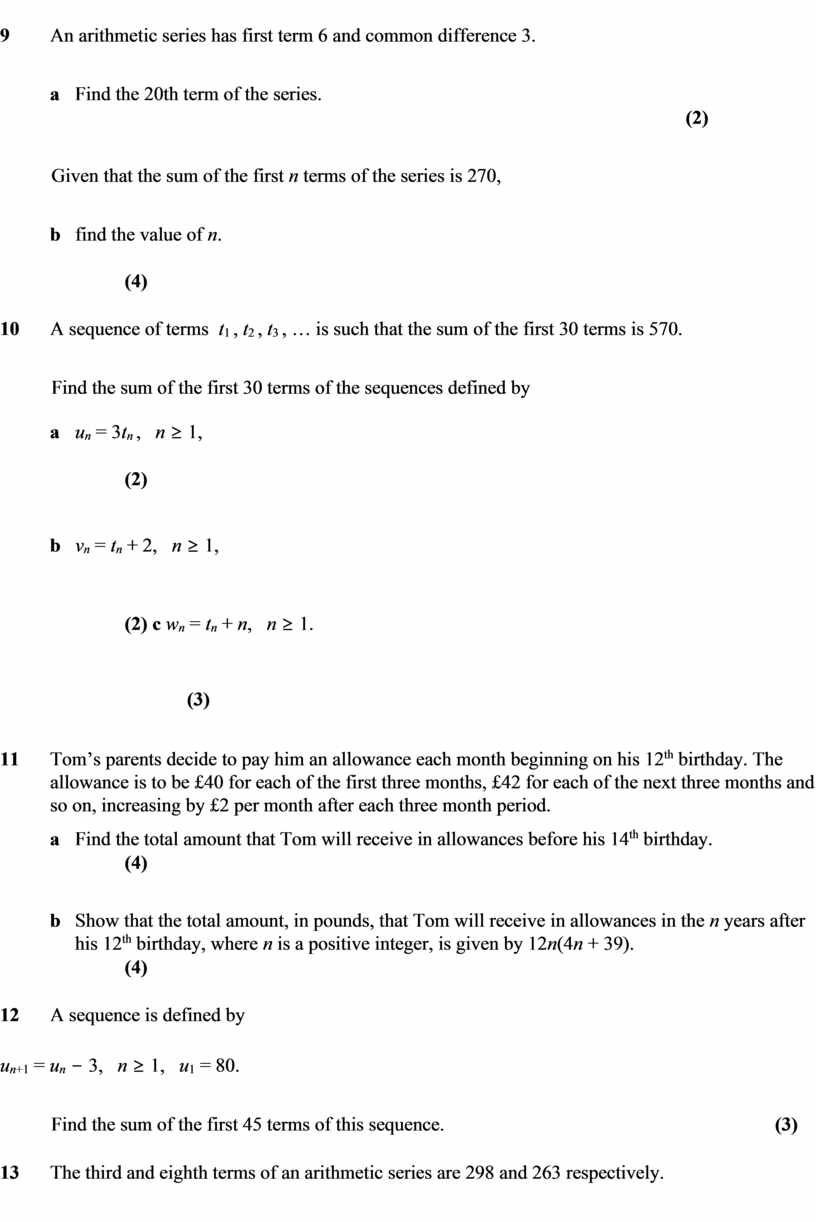C1 Sequences and Series - Questions
Оценка 4.6

# C1 Sequences and Series - Questions

Оценка 4.6
pdf
13.05.2020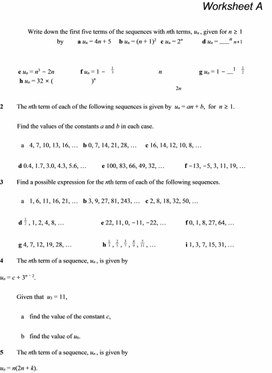C1 Sequences and Series - Questions.pdf

# Worksheet A

Write down the first five terms of the sequences with nth terms, un , given for n 1 by  a un = 4n + 5          b un = (n + 1)2   c un = 2n           d un =n n+1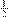e un = n3 2n               f un = 1     n              g un = 11               h un = 32 × (    )n

2n

2                The nth term of each of the following sequences is given by  un = an + b,  for  n 1.

Find the values of the constants a and b in each case.

a      4, 7, 10, 13, 16, …   b 0, 7, 14, 21, 28, …     c 16, 14, 12, 10, 8, …

d 0.4, 1.7, 3.0, 4.3, 5.6, …         e 100, 83, 66, 49, 32, …           f 13, 5, 3, 11, 19, …

3                Find a possible expression for the nth term of each of the following sequences.

a      1, 6, 11, 16, 21, …   b 3, 9, 27, 81, 243, …   c 2, 8, 18, 32, 50, …

d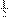, 1, 2, 4, 8, …                     e 22, 11, 0, 11, 22, …           f 0, 1, 8, 27, 64, …

g 4, 7, 12, 19, 28, …                 h,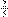,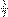,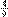,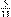, …                 i 1, 3, 7, 15, 31, …

4                The nth term of a sequence, un , is given by

un = c + 3n 2.

Given that  u3 = 11,

a      find the value of the constant c,

b      find the value of u6.

5                The nth term of a sequence, un , is given by

un = n(2n + k).

Given that  u6 = 2u4 2,

a      find the value of the constant k,

b      prove that for all values of nun un 1 = 4n + 3.

6                The nth term of a sequence, un , is given by

un = kn 3.

Given that  u1 + u2 = 0,

a find the two possible values of the constant k.

b For each value of k found in part a, find the corresponding value of u5.

7                Write down the first four terms of each sequence.

a      un = un–1 + 4,   n > 1,   u1 = 3             b un = 3un–1 + 1,   n > 1,   u1 = 2

c un+1 = 2un + 5,   n > 0,   u1 = –2                       d un = 7 – un–1,   n 2,   u1 = 5

e un = 2(5 – 2un–1),   n > 1,   u1 = –1                   f un =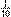(un–1 + 20),   n 2,   u1 = 10

g un+1 = 1 –un,   n 1,   u1 = 6                        h un+1 =1 ,   n 1,   u1 = 0

2 +un

 C1 SEQUENCES AND SERIES Worksheet A continued

8                In each case, write down a recurrence relation that would produce the given sequence.

a      5, 9, 13, 17, 21, …   b 1, 3, 9, 27, 81, …      c 62, 44, 26, 8, 10, …

d 120, 60, 30, 15, 7.5, …           e 4, 9, 19, 39, 79, …                 f 1, 3, 11, 43, 171, …

9                Given that the following sequences can be defined by recurrence relations of the form                   un = aun1 + bn  > 1,  find the values of the constants a and b for each sequence.

a      4, –3, –1, 3, 11, …            b 0, 8, 4, 6, 5, …          c 7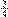, 5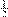, 4, 3, 2, …

10            For each of the following sequences, find expressions for u2 and u3 in terms of the constant k.

a      un = 4un–1 + 3k,   n > 1,   u1 = 1         b un+1 = kun + 5,   n > 0,   u1 = 2

c un = 4un–1k,   n > 1,   u1 = k                         d un = 2 – kun–1,    n 2,   u1 = –1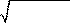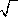e un+1 = ukn ,   n 1,   u1 = 4                                 f un+1 = 3 61k3 +un3 ,   n > 0,   u1 = k 3 3

11            A sequence is given by the recurrence relation

un =(k + 3un–1),   n > 1,   u1 = 2.

a Find an expression for u3 in terms of the constant k.

Given that  u3 = 7,

b find the value of k and the value of u4.

12            For the sequences given by the following recurrence relations, find u4 and u1.

a      un = 3un–1 – 2,   n > 1,   u3 = 10         b un+1 =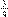un + 2,   n > 0,   u3 = 5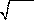c un+1 = 0.2(1 – un),   n > 0,   u3 = –0.2                d un = 12 un1 ,   n > 1,   u3 = 1

13            A sequence is defined by

un+1 = un + c,   n 1,   u1 = 2,

where c is a constant. Given that  u5 = 30,  find

a      the value of c,

b      an expression for un in terms of n.

14            The terms of a sequence  u1 , u2 , u3 , …  are given by

un = 3(un–1k),   n > 1,

where k is a constant. Given that  u1 = – 4,

a find expressions for u2 and u3 in terms of k.

Given also that  u3 = 7u2 + 3,  find

b      the value of k,

c      the value of u4.

15            A sequence of terms {tn} is defined, for n > 1, by the recurrence relation

tn = ktn–1 + 2,

where k is a constant. Given that  t1 = 1.5,

a find expressions for t2 and t3 in terms of k.

Given also that  t3 = 12,

b find the possible values of k.

# Worksheet B

For each of the following arithmetic series, write down the common difference and find the value of the 40th term.

a 4 + 10 + 16 + 22 + …             b 30 + 27 + 24 + 21 + …             c 8.9 + 11.2 + 13.5 + 15.8 + …

2                For each of the following arithmetic series, find an expression for the nth term in the form a + bn.

a 7 + 9 + 11 + 13 + …                 b+ 1+ 2+ 4+ …         c 17 + 9 + 1 + (7) + …

3                Find the sum of the first 30 terms of each of the following arithmetic series.

a 8 + 12 + 16 + 20 + …             b 60 + 53 + 46 + 39 + …                  c 7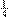+ 8+ 10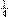+ 11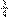+ …

4                Given the first term, a, the last term, l, and the number of terms, n, find the sum of each of the following arithmetic series.

a a = 60, l = 136, n = 20            b a = 100, l = 84.5, n = 32         c a = 28, l = 20, n = 17

5                Given the first term, a, the common difference, d, and the number of terms, n, find the sum of each of the following arithmetic series.

a a = 2, d = 9, n = 48                b a = 100, d = 5, n = 36           c a = 19, d = 13, n = 55

6                Given the first term, a, the common difference, d, and the last term, l, find the sum of each of the following arithmetic series.

a a = 8, d = 3, l = 65                 b a = 3.4, d = 1.2, l = 23.8        c a = 22, d = 8, l = 226

7                The first and third terms of an arithmetic series are 21 and 27 respectively.

a Find the common difference of the series.

b Find the sum of the first 40 terms of the series.

8                The nth term of an arithmetic series is given by 7n + 16.

Find the first term of the series and the sum of the first 35 terms of the series.

9                The second and fifth terms of an arithmetic series are 13 and 46 respectively.

a Write down two equations relating the first term, a, and the common difference, d, of the series.

b Find the values of a and d.

c Find the 40th term of the series.

10            The third and eighth terms of an arithmetic series are 72 and 37 respectively.

a Find the first term and common difference of the series.

b Find the sum of the first 25 terms of the series.

11            The fifth term of an arithmetic series is 23 and the sum of the first 10 terms of the series is 240.

a Find the first term and common difference of the series.

b Find the sum of the first 60 terms of the series.

12            a Prove that the sum of the first n natural numbers is given byn(n + 1).

b Find the sum of the natural numbers from 30 to 100 inclusive.

 C1 SEQUENCES AND SERIES Worksheet B continued

13            Write down all the terms in each of the following series summations.

5

a       (2r + 3)

r=1

9

b       (183 )r

r=1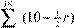10

c       (4r 1) d  r=4         r=11

14            Evaluate

20

a       (3r +1)

r=1

n

45

b       (902 )r

r=1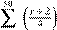30

c       (4r + 7)             d

r=3                                                               r=10

15            Given that  (4r 6) = 720,  find the value of n.

r=1

16            Find the sum of

a      all even numbers between 2 and 160 inclusive,

b      all positive integers less than 200 that are divisible by 3,

c      all integers divisible by 6 between 30 and 300 inclusive.

17            An arithmetic series has common difference 11 and tenth term 101.

a Find the first term of the series.

b Find the sum of the first 30 terms of the series.

18            The first and fifth terms of an arithmetic series are 17 and 27 respectively.

a Find the common difference of the series.

Given that the rth term of the series is 132,

b      find the value of r,

c      find the sum of the first r terms of the series.

19            The sum of the first six terms of an arithmetic series is 213 and the sum of the first ten terms of the series is 295.

a Find the first term and common difference of the series.

b Find the number of positive terms in the series.

c Hence find the maximum value of Sn , the sum of the first n terms of the series.

20            The sum, Sn , of the first n terms of an arithmetic series is given by  Sn = 2n2 + 5n.

a Evaluate S8. b Find the eighth term of the series.

c Find an expression for the nth term of the series.

21            The first three terms of an arithmetic series are (k + 2), (2k + 3) and (4k 2) respectively.

a Find the value of the constant k.

b Find the sum of the first 25 terms of the series.

22            The fifth, sixth and seventh terms of an arithmetic series are (5 t), 2t and (6t 3) respectively.

a Find the value of the constant t.

b Find the sum of the first 18 terms of the series.

# Worksheet C

The third term of an arithmetic series is 10 and the sum of the first eight terms of the series is 16.

a Find the first term and common difference of the series.

b Find the smallest value of n for which the nth term of the series is greater than 300.

2          The third and seventh terms of an arithmetic series areand 2respectively.

a Find the first term and common difference of the series.

b Show that the sum of the first n terms of the series is given by

kn(9n 5),

where k is an exact fraction to be found.

3          An arithmetic series has first term a and common difference d.

Given that the sum of the first nine terms of the series is 126,

a show that  a + 4d = 14.

Given also that the sum of the first 15 terms of the series is 277.5,

b      find the values of a and d,

c      find the sum of the first 32 terms of the series.

4                Three consecutive terms of an arithmetic series are (7k 1), (5k + 3) and (4k + 1) respectively.

a Find the value of the constant k.

b Find the smallest positive term in the series.

Given also that the series has r positive terms,              c show that the sum of the positive terms of the series is given by r(4r 3).

5                a Evaluate

30

4r .

r=1

30

i         (4r +1) ,

r=1

30

ii       (8r 5).

r=1

6                Ahmed begins making annual payments into a savings scheme. He pays in £500 in the first year and the amount he pays in increases by £40 in each subsequent year.

a Find the amount that Ahmed pays into the scheme in the eighth year.

b Show that during the first n years, Ahmed pays in a total amount, in pounds, of  20n(n + 24).

Carol starts making payments into a similar scheme at the same time as Ahmed. She pays in £400 in the first year, with the amount increasing by £60 each year.

c By forming and solving a suitable equation, find the number of years of paying into the schemes after which Carol and Ahmed will have paid in the same amount in total.

 C1 SEQUENCES AND SERIES Worksheet C continued

7                a Prove from first principles that the sum of the first n positive even numbers is  n(n + 1).

b      Find the sum of the integers between 200 and 800 that are not divisible by 4.

8                a State the formula for the sum, Sn , of the first n terms of an arithmetic series with first term a and common difference d.

b      Prove that for any arithmetic series,

S8 = 2(S6 S2). c An arithmetic series has first term 40 and common difference 3.

Find the sum of the positive terms of the series.

9                The first and fourth terms of an arithmetic series are x and (2x + 3) respectively.

a Find expressions in terms of x for

i         the seventh term of the series,

ii       the common difference of the series,

iii the sum of the first ten terms of the series.

Given also that the 20th term of the series is 52,

c      find the value of x.

10       An arithmetic series has first term a and common difference d.

Given that the sum of the first twenty terms of the series is equal to the sum of the next ten terms of the series, show that the ratio  a : d  =  11 : 2.

11            The sum, Sn , of the first n terms of a series is given by

Sn = 2n(16 n).

a Show that the sixth term of the series is 10.

b Find an expression for the nth term of the series in the form  a + bn.

c Hence, prove that the series is arithmetic.

12            A publisher decides to start producing calendars.

The publisher sells 2400 calendars during the first year of production and forecasts that the number it will sell in subsequent years will increase by 250 each year.

a      According to this forecast,

i        find how many calendars the publisher will sell during the sixth year of production,

ii      show that the publisher will sell a total of 35 250 calendars during the first ten years of production.

b      If the number of calendars sold in subsequent years increases by C each year, instead of by 250, find to the nearest unit the value of C such that the publisher would sell a total of 40 000 calendars during the first ten years of production.

13            a Prove that the sum, Sr , of the first r terms of an arithmetic series with first term a and rth term l

is given by

Sr =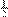r(a + l).

b The 18th term of an arithmetic series is 68 and the sum of the first 18 terms of the series is 153.

Find the first term of the series.

# Worksheet D

The second and fifth terms of an arithmetic series are 40 and 121 respectively.

 a Find the first term and common difference of the series. (4) b Find the sum of the first 25 terms of the series. (2) 2 A sequence is defined by the recurrence relation ur = ur–1 + 4,   r > 1,   u1 = 3. a Write down the first five terms of the sequence. (1) 20 b Evaluate  ∑ ur .                                                                                                                                                                                                                                     r=1 (3) 3 The first three terms of an arithmetic series are t, (2t − 5) and 8.6 respectively. a Find the value of the constant t. (2) b Find the 16th term of the series. (4) c Find the sum of the first 20 terms of the series. (2) 4 a State the formula for the sum of the first n natural numbers. (1) b Find the sum of the natural numbers from 200 to 400 inclusive. (3) c Find the value of N for which the sum of the first N natural numbers is 4950. (3) 5 A sequence of terms {un} is defined, for n ≥ 1, by the recurrence relation un+1 = k + un2, where k is a non-zero constant. Given that  u1 = 1, a find expressions for u2 and u3 in terms of k. (3) Given also that  u3 = 1, b find the value of k, (3) c state the value of u25 and give a reason for your answer. (2) 6 a Find the sum of the integers between 1 and 500 that are divisible by 3. (3) 20 b Evaluate  ∑ (5r −1) .                                                                                                                                                                                                          r=3 (3) 7 a Prove that the sum, Sn , of the first n terms of an arithmetic series with first term a and common difference d is given by

Sn =n[2a + (n 1)d].                                                                                   (4)

 b An arithmetic series has first term −1 and common difference 6. Verify by calculation that the largest value of n for which the sum of the first n terms of the series is less than 2000 is 26. (3) 8 A sequence is defined by the recurrence relation tn+1 = 4 – ktn,   n > 0,   t1 = –2, where k is a positive constant.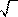Given that t3 = 3, show that k = –1 +6 .                                                                               (6)

 C1 SEQUENCES AND SERIES Worksheet D continued

9                An arithmetic series has first term 6 and common difference 3.

a      Find the 20th term of the series.                                                                                                                                                                                                                  (2)

Given that the sum of the first n terms of the series is 270,

b      find the value of n.                                                                                                                                                                                                                                                               (4)

10            A sequence of terms  t1 , t2 , t3 , … is such that the sum of the first 30 terms is 570.

Find the sum of the first 30 terms of the sequences defined by

a      un = 3tn ,   n 1,                                                                                                                                                                                                                                                                               (2)

b      vn = tn + 2,   n 1,                                                                                                                                                                                                                                                                (2) c wn = tn + n,   n 1.                                                                                                                                                                                                                                                                    (3)

11            Tom’s parents decide to pay him an allowance each month beginning on his 12th birthday. The allowance is to be £40 for each of the first three months, £42 for each of the next three months and so on, increasing by £2 per month after each three month period.

a      Find the total amount that Tom will receive in allowances before his 14th birthday.                  (4)

b      Show that the total amount, in pounds, that Tom will receive in allowances in the n years after his 12th birthday, where n is a positive integer, is given by 12n(4n + 39).                                             (4)

12            A sequence is defined by

un+1 = un 3,   n 1,   u1 = 80.

Find the sum of the first 45 terms of this sequence.                                                                  (3)

13            The third and eighth terms of an arithmetic series are 298 and 263 respectively.

a      Find the common difference of the series.                                                                                                                                                                             (3)

b      Find the number of positive terms in the series.                                                                                                                                                         (4)

c      Find the maximum value of Sn , the sum of the first n terms of the series.                                                       (3)

n

14            a Find and simplify an expression in terms of n for  (6r + 4) .                                                                                        (3)

r=1

b Hence, show that

2n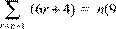n + 7).                                                                              (4)

15            The nth term of a sequence, un , is given by

un = kn n.

Given that  u2 + u4 = 6  and that k is a positive constant,

ashow that  k =            3 ,                                                                                                                                                                                                                                                                 (5)

b      show that  u3 = 3u1.                                                                                                                                                                                                                                                 (3)

16            The first three terms of an arithmetic series are (k + 4), (4k 2) and (k2 2) respectively, where k is a constant.

a      Show that  k2 7k + 6 = 0.                                                                                                                                                                                                                            (2)

Given also that the common difference of the series is positive,

b      find the 15th term of the series.                                                                                                                                                                                                                    (4)

## Given that u 6 = 2 u 4 − 2, a find the value of the constant k , b prove that for all values…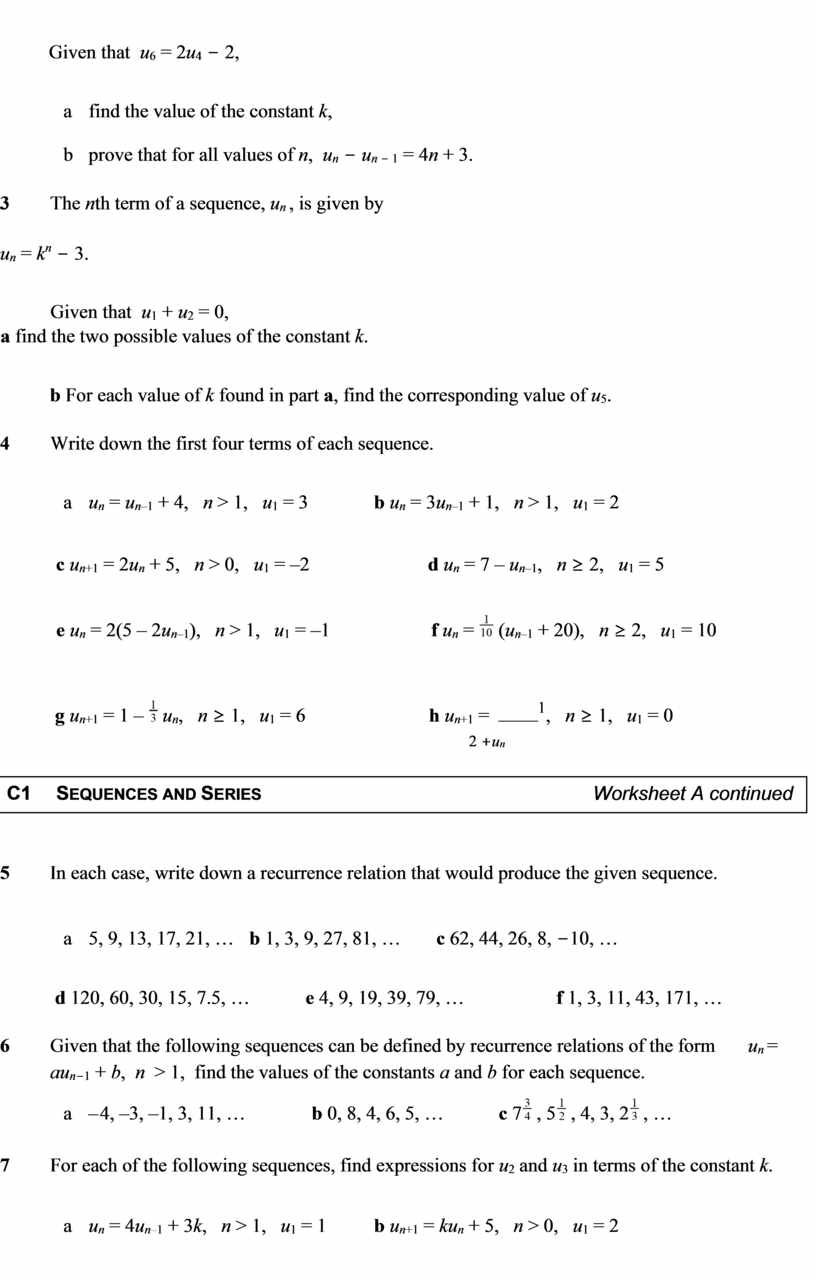## A sequence is given by the recurrence relation u n = ( k + 3 u n– 1 ), n > 1, u 1 =…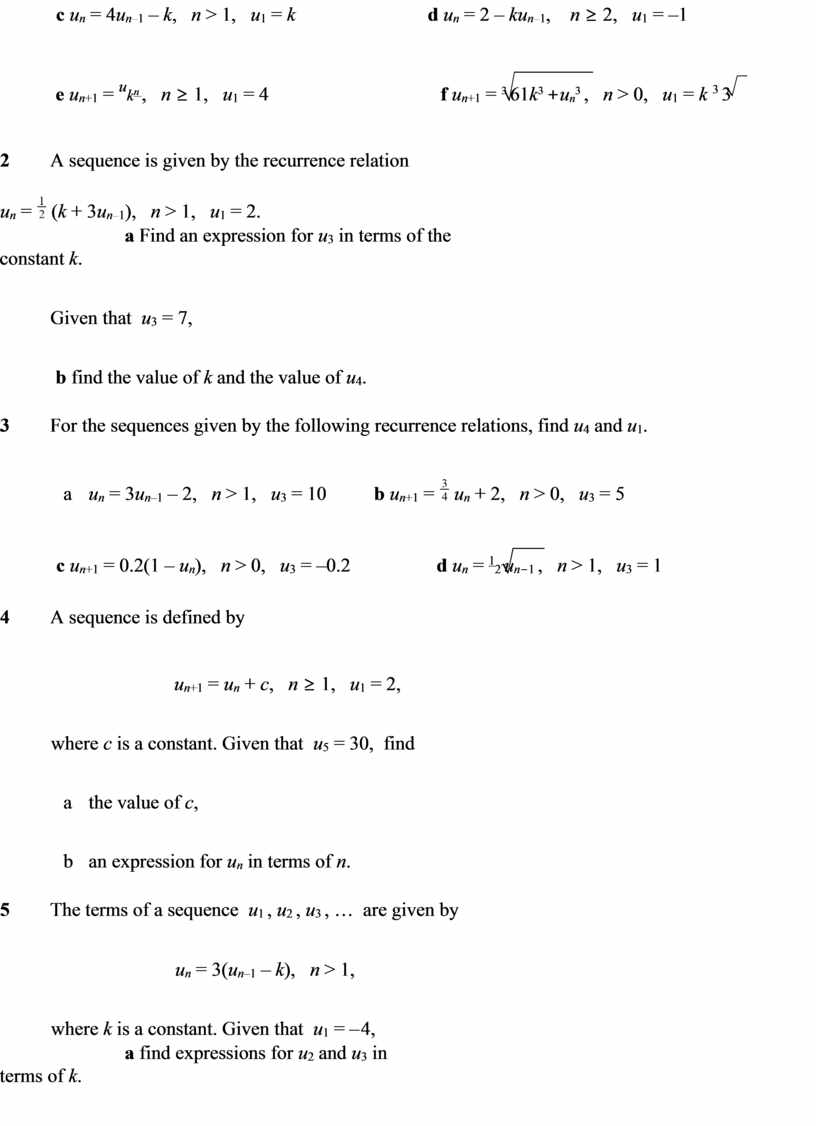## Given also that u 3 = 7 u 2 + 3, find b the value of k , c the value of u 4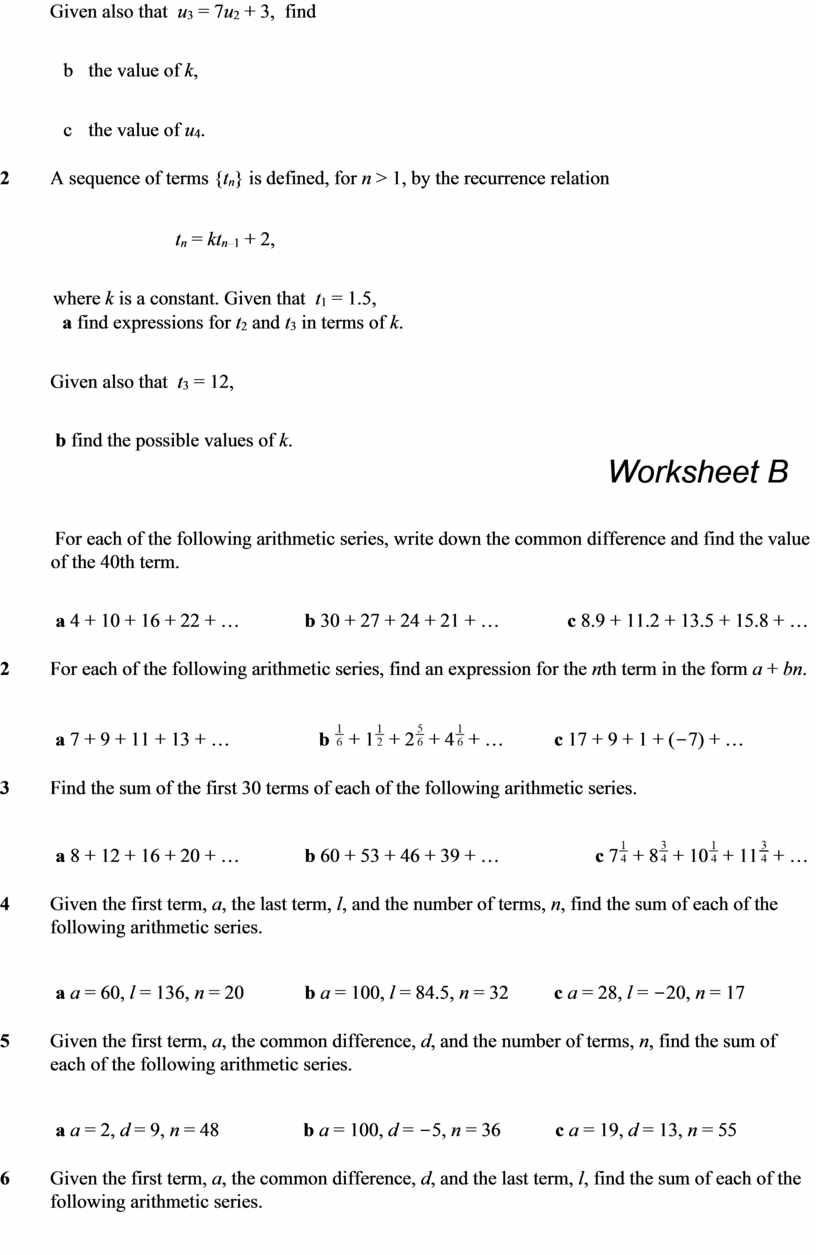## The first and third terms of an arithmetic series are 21 and 27 respectively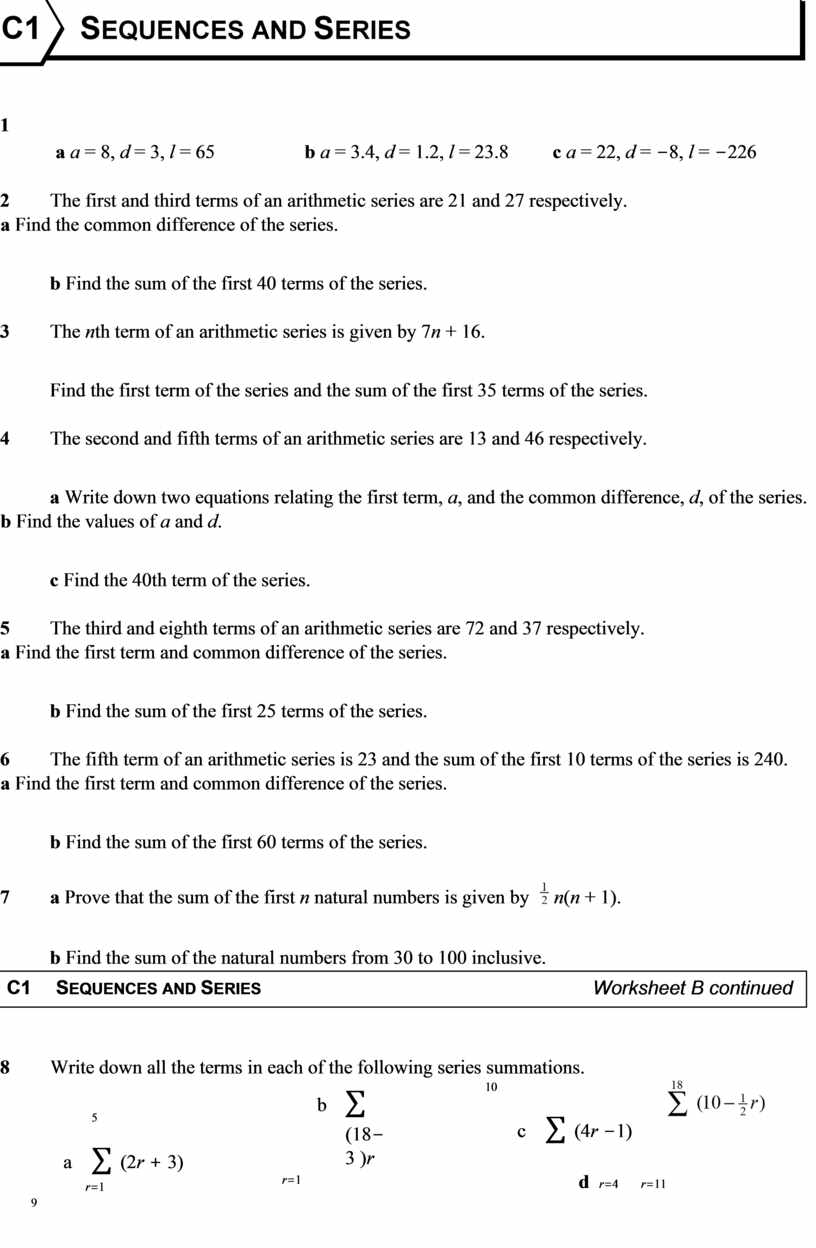## Given that ∑ (4 r − 6) = 720, find the value of n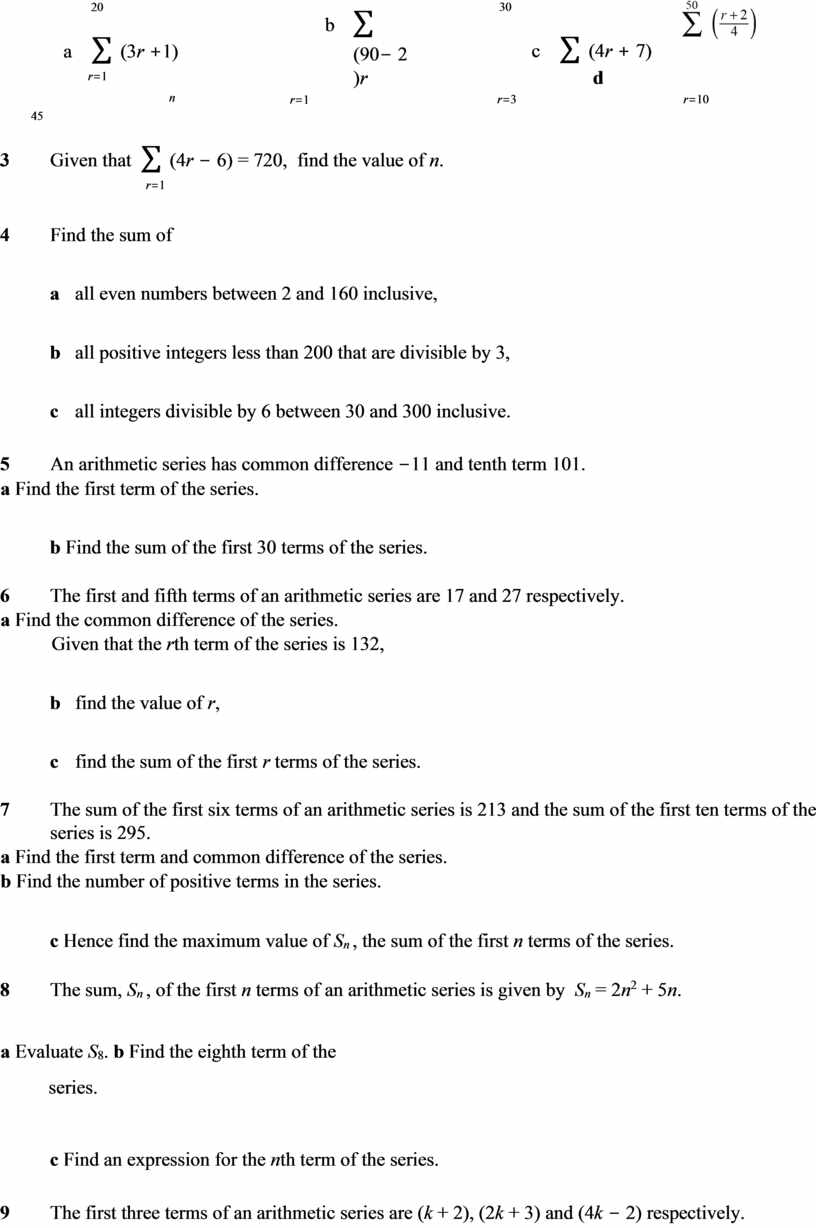## Given also that the series has r positive terms, c show that the sum of the positive terms of the series is given by r…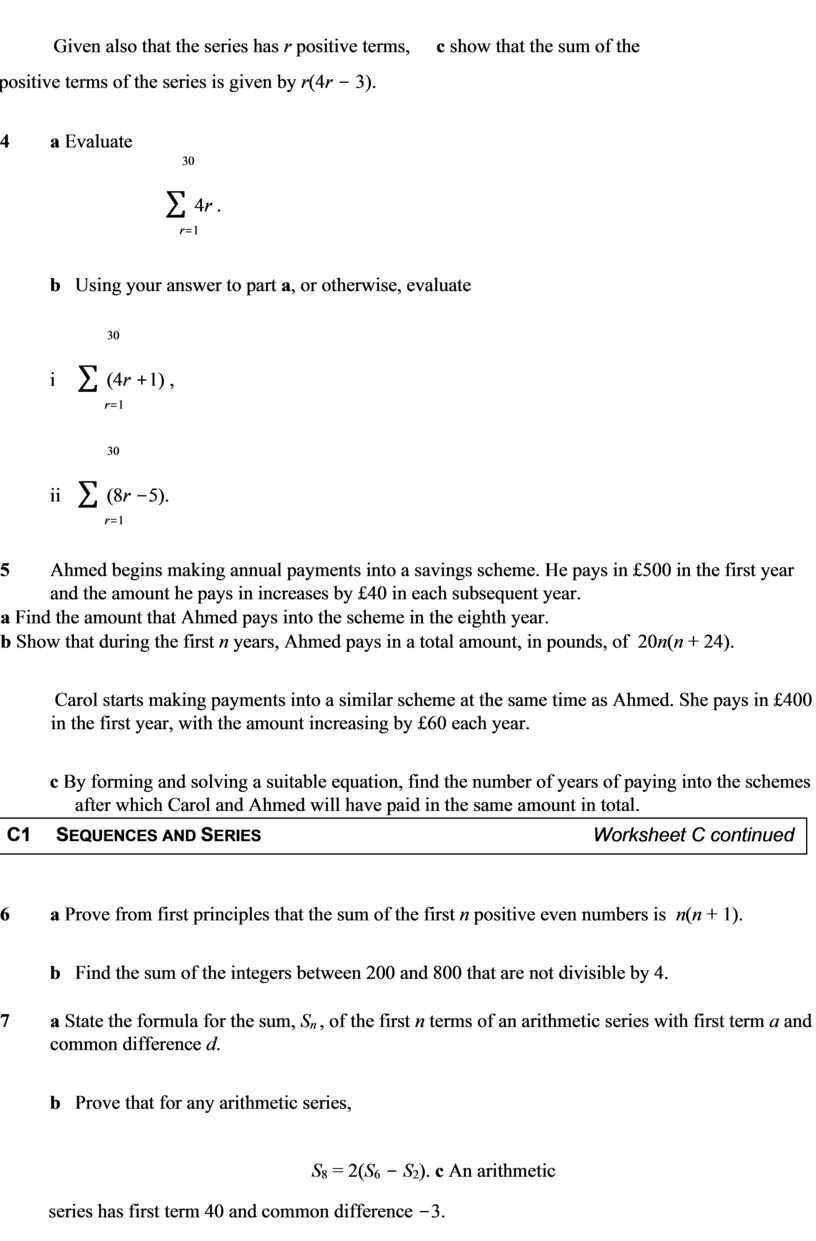## If the number of calendars sold in subsequent years increases by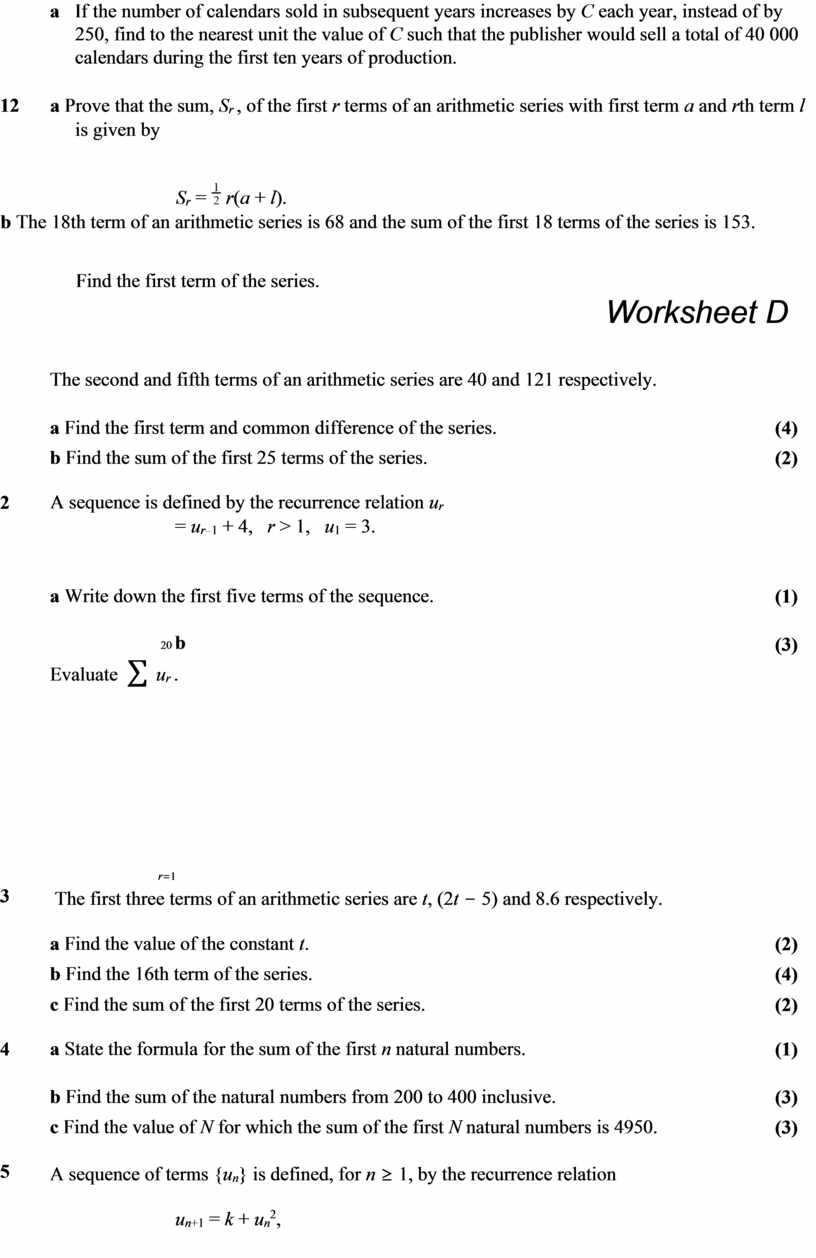## Given that u 1 = 1, a find expressions for u 2 and u 3 in terms of k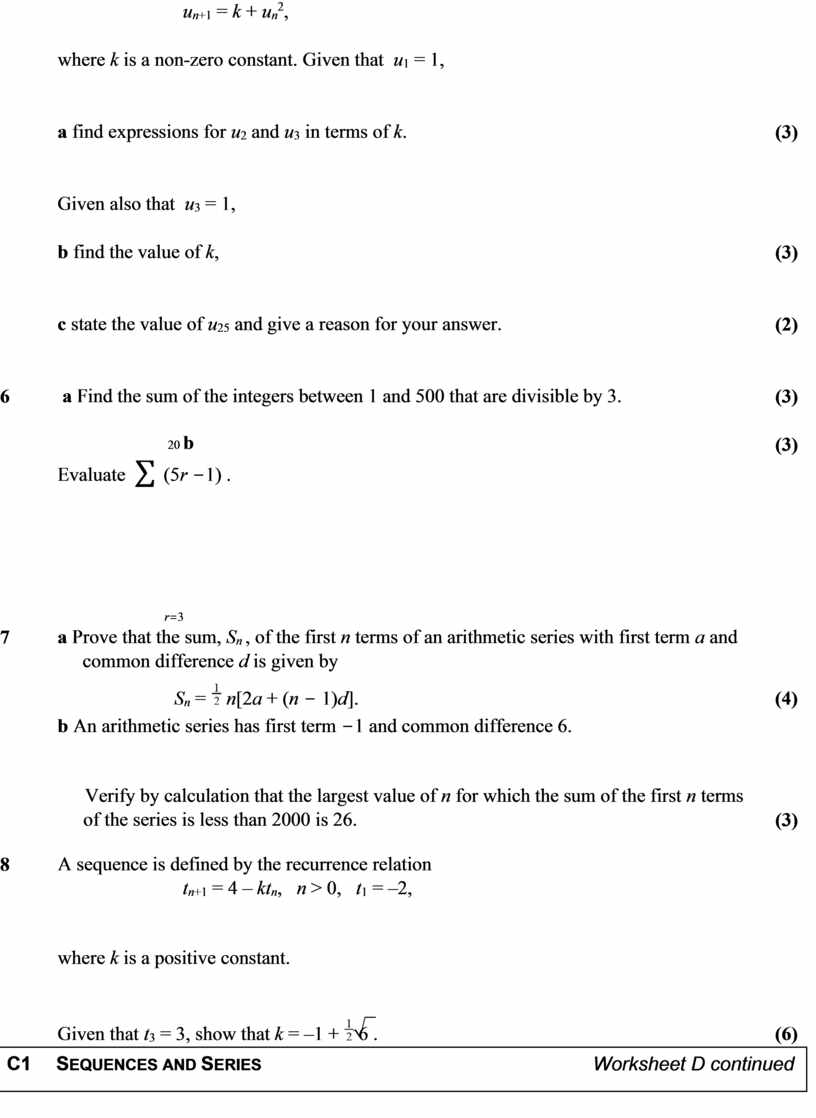## An arithmetic series has first term 6 and common difference 3